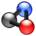# Acids and BasesThis article is part of the ChemHelp Tutoring Wiki

# Definition of Acid and Base

### The Arrhenium Theory of acids and Bases

Acid: Any substance which releases H+ in water or any ionic speicies whose formula starts with an "H"

Base: Any substance which release OH- in water; any ionic species whose formula ends with an "OH"

Salt: the neutralization product which results when an acid and bases react

### Lewis Acids and Bases

Acids: acception a pair of elections

Bases: donates a pair of electrons

# "Strong and Weak" acids and Bases

Strong acid or base is 100% in solution

Weak acid or base is less than 100% ionized solutio

# Concept of Ka and Kb

Ka: acid ionization constant Kb: base ionization constant

Ka (conjugate acid) x Kb (conjugate base) = Kw= 1.0 x 10^-14

pH= -log[H+]

pOH= -log [OH-]

pKw= pH + pOH

# concpet Neutral, Acidic and Basic

"Neutral" solution: [H3O+]= [OH-]

"Acidic" solution: [H3O+] > [OH-]

"Basic" solution: [H3O+] < [OH-]

# Concept of "Weak acid" and "Strong acid"

Strong acid or base is 100% in solution

Weak acid or base is less than 100% ionized solutio

# Predicting Acidity

-The smaller the pKa, the stronger the acid

IMPORTANT: The more stable its conjugate base, the stronger the acid

## The Strength of an acid depends on 5 factors

### 1. Size

The bigger the atom bonded to the hydrogen, the more acidic the acid.

Example: HI is a stronger acid than HF. The conjugate base of HI is I- , which is more stable than F- because the charge is spread out (it is more polarizable).

Note: size "overrides" electronegativity

### 2.Electronegativity

When the atom bonded to the hydrogen increases in electronegativity (down a row), the strength of the acid increases.

Example: CH3OH is more acidic than NH3 because Oxygen is more electronegative than Nitrogen, and they are in the same row.

### 3. Hybridization

Hybridization affects electronegativity of an atom.

Electonegativity of the atom attached to hydrogen:

``` (most "s" character)   sp > sp2 >  sp3  (least "s" character)
```

Example: HCCH is more acidic than H2C=CH2, which is more acidic than CH3CH3.

### 4. Inductive Effect

Induction or electron withdrawal refers to the shifting of electrons due to the presence of electronegative atoms nearby, causing a decrease in charge density. The more electronegative the atom and the closer it is to the acidic H, the stronger the acid.

Example: ClCH2COOH is a stronger base than CH3COOH. The conjugate bases of both compounds has resonance and delocalization of electrons. The main difference here is the presence of the Cl which "pulls" some of the electron density away from the negative charge.

### 5. Electron Delocalization/Resonance

Delocalization of electrons decreases charge density, increasing stability. An acid with a conjugate base that has delocalized electrons due to resonance is more acidic than an acid with a conjugate base with localized electrons.

Example: CH3COOH is a stronger acid than CH3OH

An acid with a pKa less than the pH of the solvent it is in, will be in it's conjugate base form

Strong acids may have a combination of these factors (for example, both inductive withdrawal and resonance)

## Buffers

Buffer solutions are solutions that resist change in Hydronium ion and the hydroxide ion concentration (and consequently pH) upon addition of small amounts of acid or base, or upon dilution. Buffer solutions consist of a weak acid and its conjugate base (more common) or a weak base and its conjugate acid (less common). The resistive action is the result of the equilibrium between the weak acid (HA) and its conjugate base (A−):

HA(aq) + H2O(l) → H3O+(aq) + A-(aq) Any alkali added to the solution is consumed by the Hydronium ions. These ions are mostly regenerated as the equilibrium moves to the right and some of the acid dissociates into Hydronium ions and the conjugate base. If a strong acid is added, the conjugate base is protonated, and the pH is almost entirely restored. This is an example of Le Chatelier's principle and the common ion effect. This contrasts with solutions of strong acids or strong bases, where any additional strong acid or base can greatly change the pH. This may be easier to see by comparing two graphs when an strong acid is titrated with a strong base the curve will have a large gradient throughout showing that a small addition of base/acid will have a large effect compared to a weak acid/strong base titration curve which will have a smaller gradient near the pKa.

Titration of a weak acid with a strong base the flat region at pKa is the buffering region. Titration of a strong acid with a strong base. Note the sharp rise in pH: this solution can not buffer.When writing about buffer systems they can be represented as salt of conjugate base/acid, or base/salt of conjugate acid. It should be noted that here buffer solutions are presented in terms of the Brønsted-Lowry notion of acids and bases, as opposed to the Lewis acid-base theory (see acid-base reaction theories). Omitted here are buffer solutions prepared with solvents other than water.

Back to ChemHelp Back to Chem154